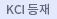#검색
다국어 입력

http://chineseinput.net/에서 pinyin(병음)방식으로 중국어를 변환할 수 있습니다.

변환된 중국어를 복사하여 사용하시면 됩니다.

예시)
• 中文 을 입력하시려면 zhongwen을 입력하시고 space를누르시면됩니다.
• 北京 을 입력하시려면 beijing을 입력하시고 space를 누르시면 됩니다.
닫기

검색결과 좁혀 보기

• 좁혀본 항목 보기순서

• 원문유무
• 음성지원유무
• 원문제공처
• 등재정보
• 학술지명
• 주제분류
• 발행연도
• 작성언어
• 저자

오늘 본 자료

• 오늘 본 자료가 없습니다.
더보기
• 무료
• 기관 내 무료
• 유료
••협착된 경동맥 내의 벽전단응력 및 혈관의 탄성적 거동

The characteristics of blood flow and the interaction between the blood vessel and blood flow play important roles in plaque cap rupture and the growth of atherosclerosis which may lead directly to a heart attack or a stroke. In this study, carotid arteries with different stenoses have been numerically simulated to investigate the wall shear stress(WSS) and the elastic motion of the vessel. Blood flow has been treated as physiological, laminar and incompressible flow. To model the shear thining behavior of the blood, the Carreau-Yasuda model has been employed but the viscoelasticity of blood has not been considered. The results show that the WSS of severe(75%) stenosis is much higher than those of 25% and 50% stenosis in the region of stenosis. With the increase in the stenosis thickness, the expansion ratio of the center of the stenosis decreases while the expansion ratio of the upstream region of the stenosis increases.

•열전 발전(TEG) 모듈의 성능 예측을 위한 수치해석적 연구

The present study develops a two-dimensional numerical code that can predict the performance of a thermoelectric generator module including a p-leg/n-leg pair and top and bottom electrodes. The present code can simulate the detailed thermoelectric phenomena including the heat flow, electric current, Joule heating, Peltier heating, and Thomson heating, together with the efficiency of the modules whose properties depend on the temperature. The present numerical code can be used for the design optimization of a thermoelectric power generator. (Received December 03, 2014)

• 이송되는 컵 형상에 따른 자유표면의 거동 특성에 관한 연구

A manipulator is operated for the motion of mechanical hands or arms. When a cup including liquid inside is shifted by a manipulator, it is important to know how a free surface of the liquid moves. In this study, non dimensional parameters have been found that affect the rise of the free surface in a cup moving with constant acceleration. The non-dimensional parameters are the dimensionless time, the ratio of inertia effect to viscous effect (the Reynolds number), aspect ratio of the liquid inside the cup and the acceleration ratio (the Froude number). Through this study, the height of the free surface rise in a cup has been predicted and the detailed velocities in the liquid have been examined. Generally, the maximum rise of the free surface is dependent on the Reynolds number and Froude number strongly, but on the aspect ratio weakly. However, the influence of the aspect ratio on the maximum rise of the free surface is not negligible in the range of 10 < Re < 100.

•이송되는 컵 내부의 자유 표면의 거동 특성에 대한 수치해석

A manipulator is operated for the motion of mechanical hands or arms. When a cup including liquid inside is shifted by a manipulator, it is important to know how a free surface of the liquid moves. In this study, non dimensional parameters have been found that affect the rise of the free surface in a cup moving with constant acceleration. The nondimensionalparameters are the dimensionless time, the ratio of inertia effect to viscous effect (the Reynolds number), the aspect ratio of the liquid inside the cup and the acceleration ratio (the Froude number). Through this study, the height of the free surface rise in a cup has been predicted and the detailed velocities in the liquid have been examined. Generally, the maximum rise of the free surface is dependent on the Reynolds number and Froude number strongly, but on the aspect ratio weakly. However, the influence of the aspect ratio on the maximum rise of the free surface is not negligible in the range of 10 < Re < 100.

• 운반되는 컵 내부의 액체 거동 특성에 대한 수치해석

A manipulator is operated for the motion of mechanical hands or arms. When a cup including liquid inside is shifted by a manipulator, it is important to know how a free surface of the liquid moves. In this study, non-dimensional parameters have been found that affect the rise of the free surface in a cup moving with constant acceleration. The non-dimensional parameters are the dimensionless time, the ratio of inertia effect to viscous effect (the Reynolds number), aspect ratio of the liquid inside the cup and the acceleration ratio (the Froude number). Through this study, the height of the free surface rise in a cup has been predicted and detailed velocities in the liquid have been examined. Generally the maximum rise of the free surface is dependent strongly on the Reynolds number and Froude number strongly, but on the aspect ratio weakly. But the influence of the aspect ratio on the maximum rise of the free surface in not negligible in the range of 10<Re<100.

•